# Ritz method

(diff) ← Older revision | Latest revision (diff) | Newer revision → (diff)

A method for solving problems in variational calculus and, in general, finite-dimensional extremal problems, based on optimization of a functional on finite-dimensional subspaces or manifolds.

Let the problem of finding a minimum point of a functional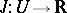on a separable Banach spacebe posed, whereis bounded from below. Let some system of elements, complete in(cf. Complete system), be given (a so-called coordinate system). In the Ritz method, the minimizing element in the-th approximation is sought in the linear hull of the firstcoordinate elements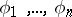, i.e. the coefficientsof the approximation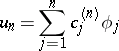are defined by the condition thatbe minimal among the specified elements. Instead of a coordinate system one can specify a sequence of subspaces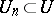, not necessarily nested.

Letbe a Hilbert space with scalar product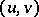, letbe a self-adjoint positive-definite (i.e.: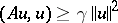for all), possibly unbounded, operator in, and let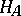be the Hilbert space obtained by completing the domain of definition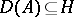ofwith respect to the norm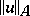generated by the scalar product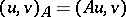,. Let it be required to solve the problem(1)

This is equivalent to the problem of finding a minimum point of the quadratic functionalwhich can be written in the formwhereis a solution of equation (1). Let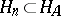,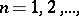be closed (usually, finite-dimensional) subspaces such that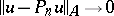asfor every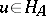, where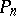is the orthogonal projection inprojecting onto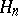. By minimizinginone obtains a Ritz approximationto the solution of equation (1); moreover,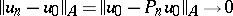as. If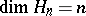and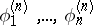is a basis in, then the coefficients of the element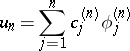(2)

are determined from the linear system of equations(3)

One can also arrive at a Ritz approximation without making use of the variational statement of the problem (1). Namely, by defining the approximation (2) from the condition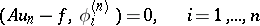(the Galerkin method), one arrives at the same system of equations (3). That is why the Ritz method for equation (1) is sometimes called the Ritz–Galerkin method.

Ritz's method is widely applied when solving eigenvalue problems, boundary value problems and operator equations in general. Letandbe self-adjoint operators in. Moreover, letbe positive definite,be positive,, and let the operator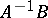be completely continuous in(cf. Completely-continuous operator). By virtue of the above requirements,is self-adjoint and positive in, and the spectrum of the problem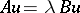(4)

consists of positive eigenvalues: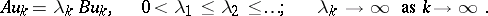Ritz's method is based on a variational determination of eigenvalues. For instance,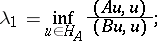by carrying out minimization only over the subspaceone obtains Ritz approximations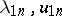of. Ifis, as above, a basis in, then the Ritz approximations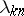of,, are determined from the equation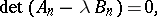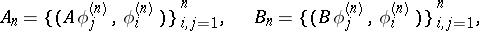and the vector of coefficientsof the approximationto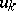is determined as a non-trivial solution of the linear homogeneous system. The Ritz method provides an approximation from above of the eigenvalues, i.e.,. If the-th eigenvalue of problem (4) is simple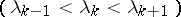, then the convergence rate of the Ritz method is characterized by the following relations: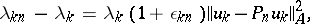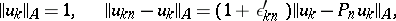where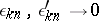as. Similar relations can be carried over to the case of multiple, but then they need certain refinements (see ). W. Ritz  proposed his method in 1908, but even earlier Lord Rayleigh had applied this method to solve certain eigenvalue problems. In this connection the Ritz method is often called the Rayleigh–Ritz method, especially if one speaks about solving an eigenvalue problem.

How to Cite This Entry:
Ritz method. Encyclopedia of Mathematics. URL: http://encyclopediaofmath.org/index.php?title=Ritz_method&oldid=19210
This article was adapted from an original article by G.M. Vainikko (originator), which appeared in Encyclopedia of Mathematics - ISBN 1402006098. See original article